# Simple Maths Multiplication Sums for KidsIn this article, we shall explore different varieties of Multiplication Sums. Multiplication is considered an essential operation of simple Arithmetical problems. Here, let us look at various types of multiplication questions.

For new school goers, multiplication is one of the basic functions of Mathematics wherein they need to multiply two different numbers to derive the third number as the final result. Kids are made to practice and solve simple multiplication sums initially so that they understand what comprises basic multiplication.

Kids are taught simple multiplication sums like remembering easy multiplication tables from 2 to 20 and solve basic Maths multiplication questions like (2×3= 6).

Here are some multiplication questions and answers that young school goers can refer to while studying the multiplication section of the subject:

Multiplication sums of two single-digit numbers: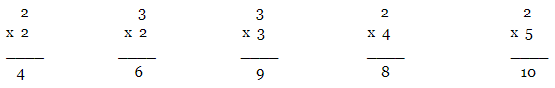Multiplication sums of one single-digit number and one double-digit number:

Steps to solve the multiplication of one single-digit number and one double-digit number.

Example: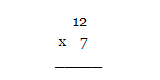Step 1: Multiply the ones place digits 2 x 7 = 14. As this is larger than 9 so keep 1 above the tens place digit and place 4 below the line of ones place digit.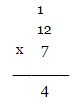Step 2: Multiply the tens place number with 7 i.e. 7 x 1 = 7 and add this with 1 which is placed over the tens digit i.e. 7 + 1 = 8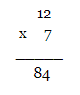Some more multiplication sums:-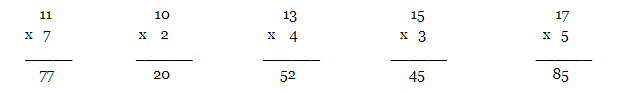Multiplication of two double-digit numbers:

Steps to solve the multiplication of two double-digit numbers.

Example: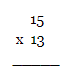Step 1: Multiply the ones place digits 3 x 5 = 15. As this is larger than 9 so keep 1 above the tens place digit and place 5 below the line of ones place digit.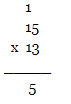Step 2: Multiply the tens place number with 3 i.e. 3 x 1 = 3 and add this with 1 which is placed over the tens digit i.e. 3 + 1 = 4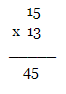Step 3: Now the number becomes 45 under the line. Then multiply the tens place number of 13 with ones place of 15 i.e. 1 x 5 = 5. Place a “0” under the ones place of 45 and place 5 under the tens place.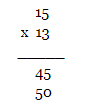Step 4: Multiply the tens place number of 13 with tens place of 15 i.e. 1 x 1 = 1. Place 1 next to 5.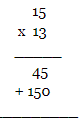Step 5: Add both the numbers under the line to get the final result.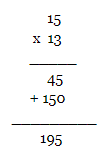Some more multiplication sums: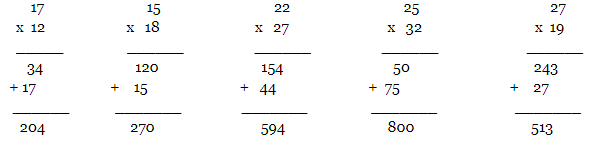Multiplication of one double-digit number and one triple-digit number: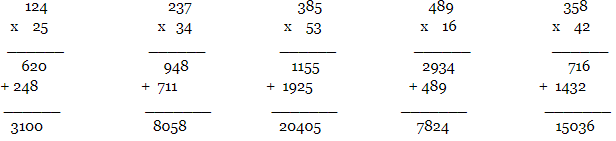## Resources for Kids

Listed below are the class-wise Maths pages for your child:

Kids learn to solve simple multiplication sums from a very young age. Some of these multiplication questions include finding the product of single and double-digit numbers, learning multiplication times tables and long multiplication questions, etc.

For more such interesting resources such poems, essays, enchanting stories for kids, fun trivia questions for kids, NCERT Solutions, etc. feel free to check our kids learning section and make learning a fun-filled experience for your child.